News: Follow us on Twitter## The All New FractalForums is now in Public Beta Testing! Visit FractalForums.org and check it out!

 Pages:    Go DownAuthor Topic: Chaosbrot type c2 improved (new type again, awesome variety)  (Read 3954 times) Description: 0 Members and 1 Guest are viewing this topic.
M Benesi
Fractal SchemerPosts: 1075« on: June 08, 2010, 09:15:28 AM »

Formula will be up tomorrow.  Formula at bottom of post...   For now, a few small random pics as it's late.

First, a 5th order "deep" zoom (11 or 12 iterations) to some sandstone cliffs at sunset: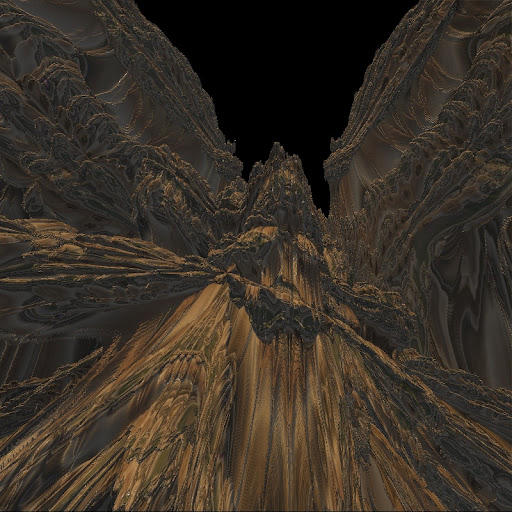2nd order rotated 90 degrees (straight on isn't interesting until 4th or 5th order, or maybe I didn't zoom enough), this resembles type D2 a bit, except when you zoom and increase iterations it is far more complex: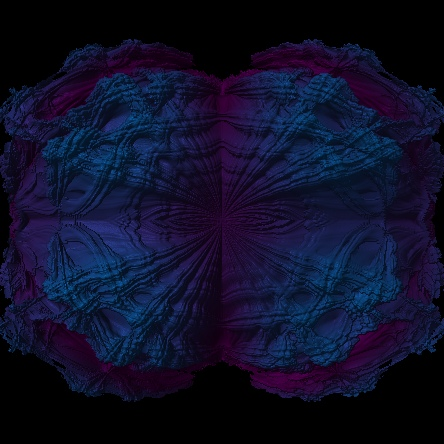5th order rotated 90 (picked random "full" shots):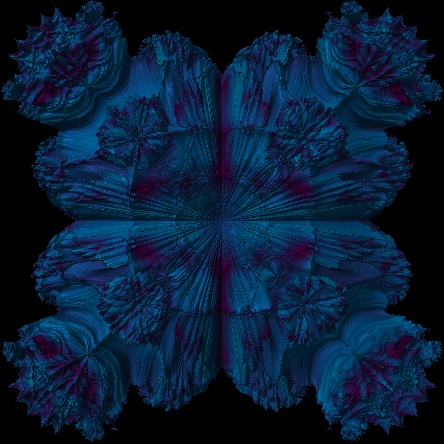6th straight on (developing complexity even for straight on shot):10th order rotated 90... different straight on though.  The odds (3,5,7,9,11...) might be same straight and rotated, or maybe its 5,9,13... dunno, but the evens seem to be different when rotated: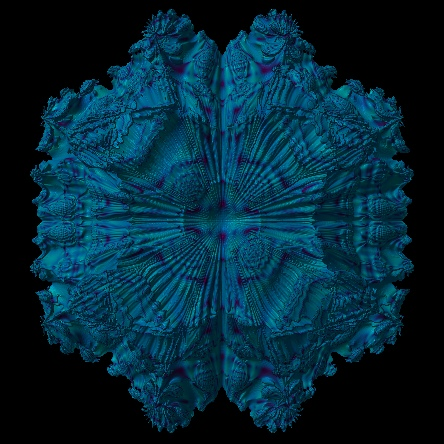Those are the previews.   Pretty simple, it's a corrected rewrite of type C2 in the benesi set.

Code:
This code is simplified (eliminated a few options which make the fractal even more chaotic, will probably put them in updated benesi set):

r2= (sqr(sx)+sqr(sx2)+sqr(sy)+sqr(sy2)+sqr(sz)+sqr(sz2))^(n/2);

// setting r2= (sx^2+sy^2+sz^2) ^ (n/2) gives a very mandelbrotesque fractal when you don't take the absolute values of pixels
// however it doesn't result in as... beautiful fractals (in my opinion) for lower z^n, of course you decide for yourself

theta=atan2(sx+flip(sy));
tango=atan2(sx2+flip(sz));
whiskey=atan2(sy2+flip(sz2));

sx=r2*cos(theta*v)+(pixelr);   //julia mode not implemented in this quick write up- haven't tried it yet
sx2=r2*cos(tango*v)+(pixelr);   //
sy=r2*sin(theta*v)+abs(pixeli);       //
sy2=r2*cos(whiskey*v)+abs(pixeli);   //    set abs for the y components.... just like a real 2d mandy y is reflected which eliminates distortions
sz=r2*sin(tango*v)+(pixelj);        //    in the z^n with even n...
sz2=r2*sin(whiskey*v)+(pixelj);   //

bail=abs(sx)+abs(sy)+abs(sz)+abs(sx2)+abs(sy2)+abs(sz2);

And here is a quick bit of the type B1 correction (it works decently, but is distorted due to imbalanced variables (2 x, 1 y, and 1 z instead of C2's balanced 2 x, 2 y, and 2 z variables)... You can correct distortion with a scalar (.5 seems fine if you multiply sx and sx2 before taking (.5*(sx^2+sx2^2)+sy+sz)^(n/2)), but I think Type C2, while not as immediately pretty as type B1, is the more chaotic, interesting, and balanced fractal (especially after z^5: a few fractally sections in z^2, more as you increase n above 5, gets more interesting as you increase it beyond 10...).  Over all it's up to you, but c2 is crazy.

Also, I believe (must recheck a bit) C2 has more variety: in the 11th order, type b1 has 4 symmetric sides, plus top/bottom symmetry, type c2 has 3 different "types" of sides (each having a matching opposite).

UPDATED VERSION BELOW THIS ONE... TYPE B3D (the D is added because for some reason I can't use type B3 without getting an error).

Code:

if (r2mode) {
r2=(sqr(sx)+sqr(sy)+sqr(sz))^(n/2);  // this is for the less distorted mandy, of course it sacrifices y/z symmetry
} else {
r2=(sqr(sx)+sqr(sx2)+sqr(sy)+sqr(sz))^(n/2);  //  distorted, but y/z symmetry intact.... you decide
}

theta=atan2(sx+flip(sy));
tango=atan2(sx2+flip(sz));

nx=r2*cos(theta*v);
nx2=r2*cos(tango*v);

ny=r2*sin(theta*v);
nz=r2*sin(tango*v);

if (juliaMode) {
sx=nx+cr;
sx2=nx2+cr;
sy=ny+ci;
sz=nz+cj;
} else {
sx=   nx + pixelr;
sx2= nx2 + pixelr;
sy=  ny +abs(pixeli);  //actually don't think absolute value effects it... should check however :D
sz=  nz +abs(pixelj);
}
z=quaternion (sx,sy,sz,0);

bail=abs(sx)+abs(sy)+abs(sz)+abs(sx2);

Use r2mode for the more (not quite perfect... but as close as I've seen) Mandelbroty version.  It even has fractallyness in seahorse valley!  (all other 3d "mandys" aren't as fractally in that area... AFAIK at the moment)

UPDATE OF TYPE B1:  TYPE B3D:
Code:
if (r2mode) {
r2=(sqr(sx)+sqr(sy)+sqr(sz))^(n/2);
r3=(sqr(sx2)+sqr(sy)+sqr(sz))^(n/2);
} else {
r2=(sqr(sx)+sqr(sx2)+sqr(sy)+sqr(sz))^(n/2);
}

theta=atan2(sx+flip(sy));
tango=atan2(sx2+flip(sz));

if (r2mode) {
if (pixelr>0) {
nx=r3*cos(theta*v);
} else {
nx=r2*cos(theta*v);
}
if (pixelr>0) {
nx2=r2*cos(tango*v);
} else {
nx2=r3*cos(tango*v);
}
ny=r2*sin(theta*v);
nz=r3*sin(tango*v);
} else {
nx=r2*cos(theta*v);
nx2=r2*cos(tango*v);
ny=r2*sin(theta*v);
nz=r2*sin(tango*v);
}
if (juliaMode) {
sx=nx+cr;
sx2=nx2+cr;
sy=ny+ci;
sz=nz+cj;
} else {
sx=nx+(pixelr);
sx2=nx2+(pixelr);
sy=ny+abs(pixeli);
sz=nz+abs(pixelj);
}
z=quaternion (sx,sy,sz,sx2);

if (bailcontrol) {
bail=abs(sx)+abs(sy)+abs(sz)+abs(sx2);
} else {
bail=abs(sx)+abs(sy)+abs(sz);
}

}

 « Last Edit: June 10, 2010, 11:01:47 PM by M Benesi »Logged

M Benesi
Fractal SchemerPosts: 1075« Reply #1 on: June 10, 2010, 09:24:42 AM »

All right, here are a few images from both types.

First, a bit of babble:  I initially thought that type C2 had greater variety: more faces than the b1.  Well, apparently it does for certain z^n.  For z^5,9,13,17..... it has symmetry all around.  For even z^n, it always lacks complete symmetry (like a regular Mandelbrot type).  The apparent lack of symmetry for powers 3,7,11,13.... may be due to the fact that it simply has a slightly off kilter layout.

Also, I was looking through the original super long mandelbulb thread, and noticed that the z^2 of type b1 looks a LOT like David Makin's "true mandy".  So we now have an additional form of math to play with (rotational stuff).  Nice to be able to develop new methods to generalize rotations.... or discover old ones that someone else already knows.Anyways, here are a few C2, followed by a couple pretty B1s.  The B1 is easier to color with orbital coloring due to the fact that it doesn't have as many variables.  I'll have to figure something out for c2 coloring, but for now it's ramshackle at best.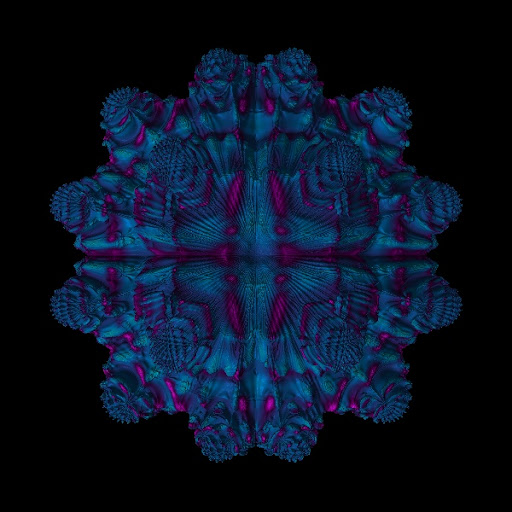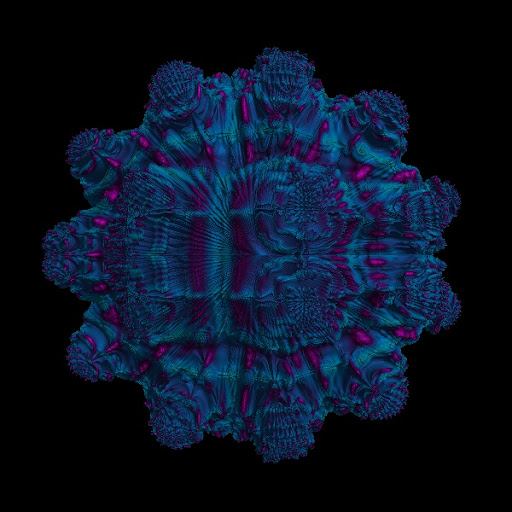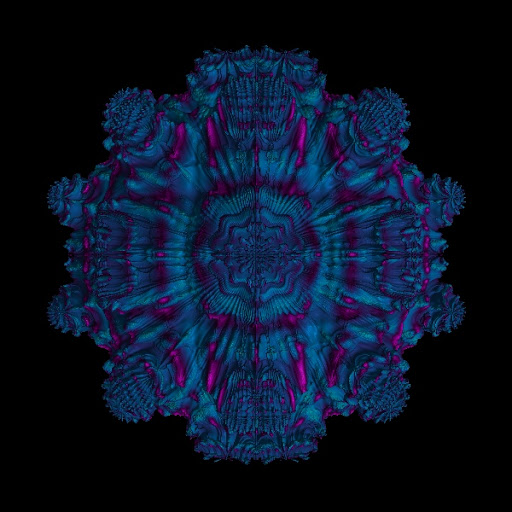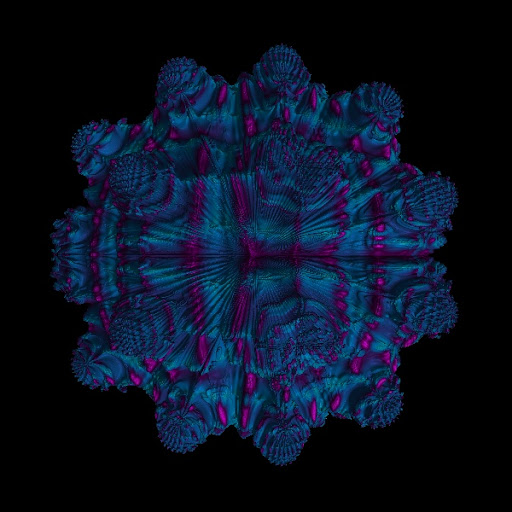I think this is the z^13.. the above are 12s.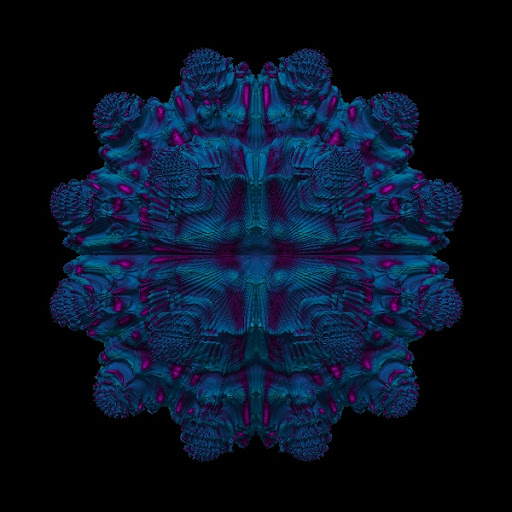these are 13s: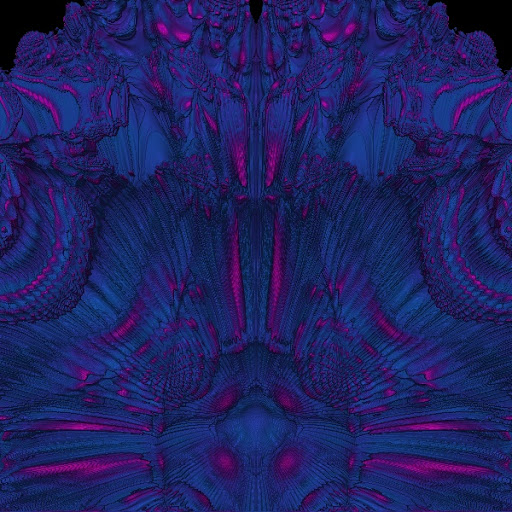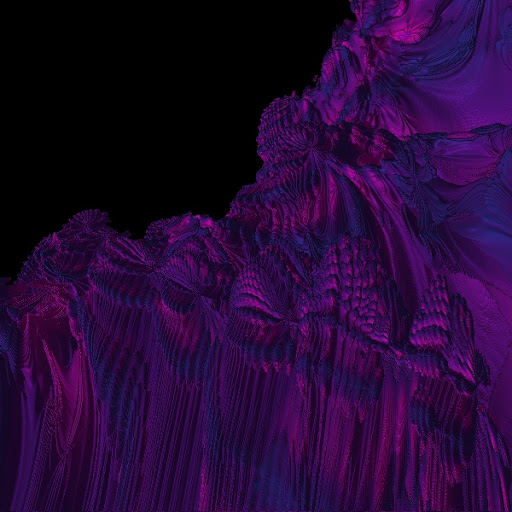here are the B1s: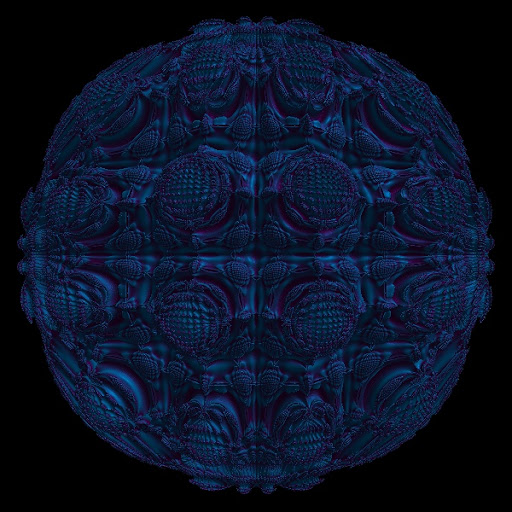Notice the distortion in this one.  It's due to uneven variable distribution (which C2 corrects).  We can use a scalar to deal with it, discover the math to correct it, or just enjoy this types natural beauty...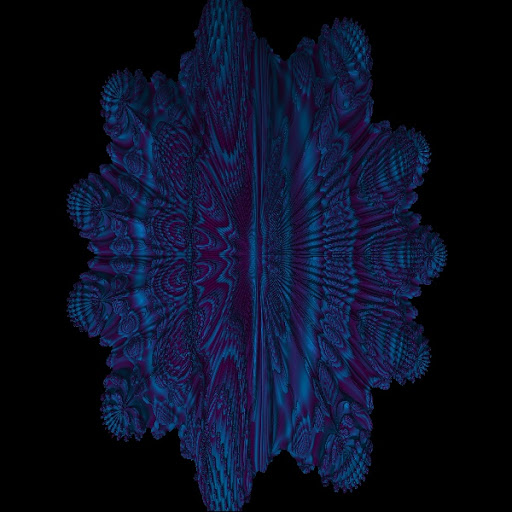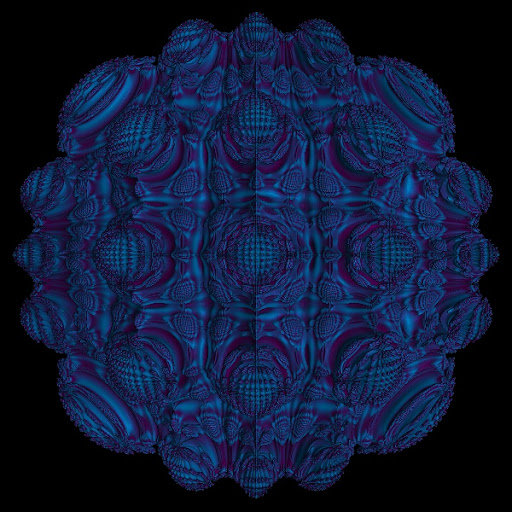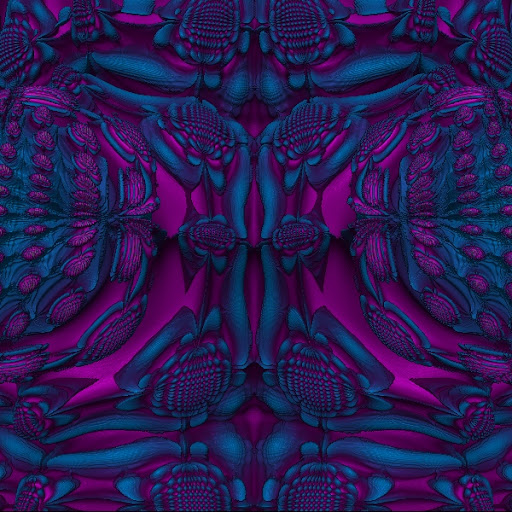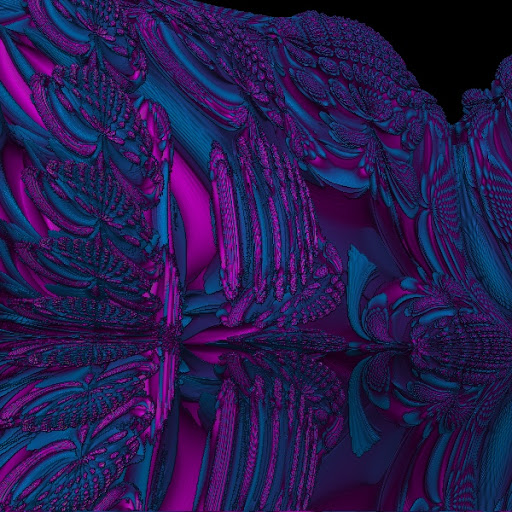Logged

 Pages:    Go Down
 Related Topics Subject Started by Replies Views Last post3D Mandelbrot type z^3 + p 3D Fractal Generation Buddhi 4 4002July 20, 2009, 04:17:50 AM by lycium3d Fractal Type that works for z^2 3D Fractal Generation M Benesi 10 4087February 17, 2010, 09:42:23 PM by M Benesia set of 4 dimensional type d2 fractals Images Showcase (Rate My Fractal) M Benesi 1 1484April 23, 2010, 11:05:15 AM by Power 8type e (new type.. modified xyz vs mag formula) Images Showcase (Rate My Fractal) M Benesi 0 2507May 19, 2010, 09:51:09 AM by M BenesiNew fractal type... latest 3d type.. a z^2 for Benoit Images Showcase (Rate My Fractal) M Benesi 0 5631October 21, 2010, 07:14:00 AM by M Benesi